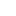# Unit 3: Elasticity

## Introduction

What’s in This Chapter? In Unit 2, we learned that if the price of a product increases, the amount demanded decreases. But how much does it decrease? The “how much” describes the concept of price elasticity of...

## Section 1: Demand Curves and Elasticity

Price Elasticity of Demand Price elasticity of demand measures the responsiveness of buyers to a price change. If the price of gasoline increases by 10%, how will this affect the amount of gasoline purchased? Will the amount...

## Section 2: Elasticity and the Slope of the Demand Curve

Demand Curves and Elasticity Elasticity affects the slope of a product’s demand curve. A greater slope means a steeper demand curve and a less-elastic product. In the graph below, the steeper demand curve, D1, shows a...

## Section 3: Determinants of Price Elasticity of Demand

Elasticity Determinants Some products are elastic (buyers are price sensitive), and some products are inelastic (buyers are not price sensitive). What makes people more sensitive to one product’s price change compared to...

## Section 4: Elasticity and Total Revenue

Definition of Elastic, Inelastic, and Unit Elastic Demand  By definition: 1. A product is elastic when its elasticity is greater than 1. When a product is elastic and its price changes, the percentage change in quantity demanded...

## Section 5: Income Elasticity of Demand, Cross Price Elasticity of Demand, and Price Elasticity of Supply

Income Elasticity of Demand Income elasticity of demand measures the percentage change in a buyer’s purchase of a product as a result of a percentage change in her/his income. So income elasticity of demand is ei = the...